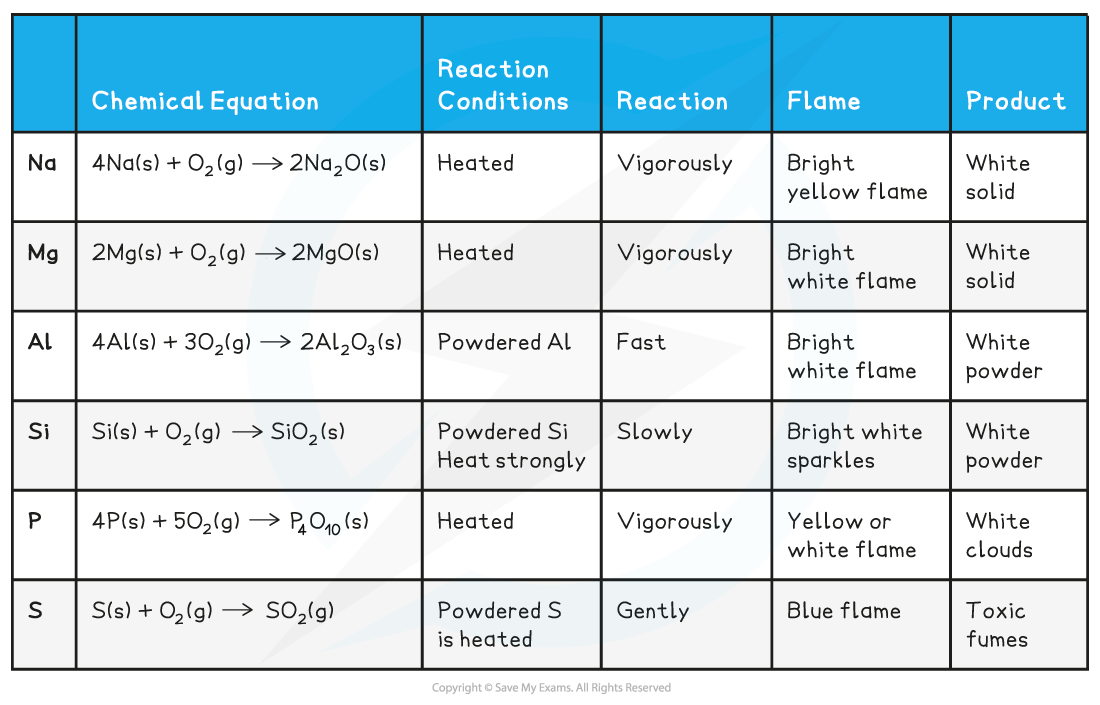# AQA A Level Chemistry复习笔记6.1.2 Reaction with Oxygen

### Reaction with Oxygen

#### Oxide Formation

• The period 3 elements, excluding chlorine and argon, combine with oxygen to form oxides
• The oxide formed will contain the elements in their highest oxidation state
• The following equations show these reactions:

4Na (s) + O2 (g) → 2Na2O (s)

2Mg (s) + O2 (g) → 2MgO (s)

4Al (s) + 3O2 (g)  → 2Al2O3 (s)

Si (s) + O2 (g)→ SiO2 (s)

4P (s) + 5O2 (g) → P4O10 (s)

S (s) + O2 (g) → SO2 (g)

Reaction of Period 3 elements with oxygen table• Sulfur can actually form two oxides - SO2 and SO3
• For SO3 to form, a catalyst must be used and the reaction must take place at a very high temperature
• The equation for this reaction is:

2S (s) + 3O2 (g) → 2SO3 (g)

• The oxides formed from these reactions have different physical properties, depending on the type of structure and bonding
• Sodium oxide, magnesium oxide and aluminium oxide are all ionic oxides
• Sodium, magnesium and aluminium are all metals and oxygen is a non-metal

• Silicon oxide has a giant covalent structure, like diamond
• Phosphorus oxide and sulfur dioxide are simple covalent molecules